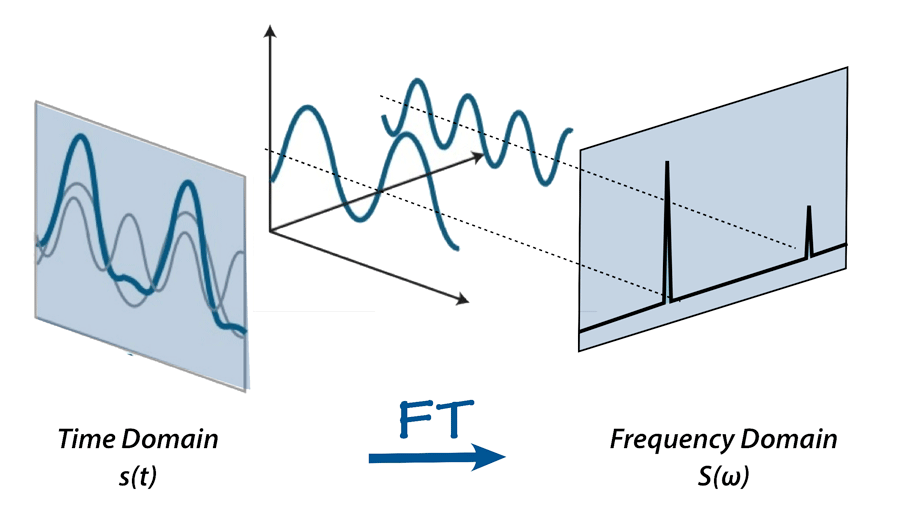/  Top Machine learning interview questions and answers   /  What do you mean by Fourier Transforms? How can we use in Machine Learning?## What do you mean by Fourier Transforms? How can we use in Machine Learning?

Fourier Transforms means converting or decomposes a signal into frequencies. Fourier Transform moves from Time domain to Frequency domain. If we want to move from Frequency domain to Time domain, we can do it by Inverse Fourier Transform.

Fourier transform is widely used not only in signal (radio, acoustic, etc.) processing but also in image analysis e.g. edge detection, image filtering, image reconstruction, and image compression.

Fourier transform of your data can expand accessible information about the analyzed sample.

```>>> from scipy.fftpack import fft

>>> # Number of sample points

>>> N = 600

>>> # sample spacing

>>> T = 1.0 / 800.0

>>> x = np.linspace(0.0, N*T, N)

>>> y = np.sin(50.0 * 2.0*np.pi*x) + 0.5*np.sin(80.0 * 2.0*np.pi*x)

>>> yf = fft(y)

>>> from scipy.signal import blackman

>>> w = blackman(N)

>>> ywf = fft(y*w)

>>> xf = np.linspace(0.0, 1.0/(2.0*T), N//2)

>>> import matplotlib.pyplot as plt

>>> plt.semilogy(xf[1:N//2], 2.0/N * np.abs(yf[1:N//2]), '-b')

>>> plt.semilogy(xf[1:N//2], 2.0/N * np.abs(ywf[1:N//2]), '-r')

>>> plt.legend(['FFT', 'FFT w. window'])

>>> plt.grid()

>> plt.show()

```